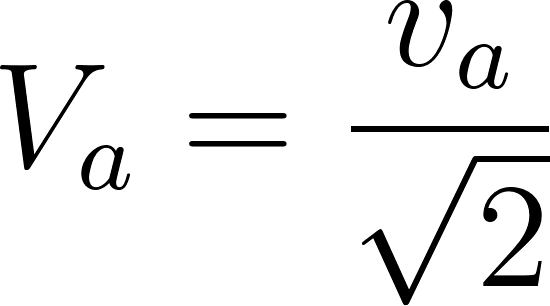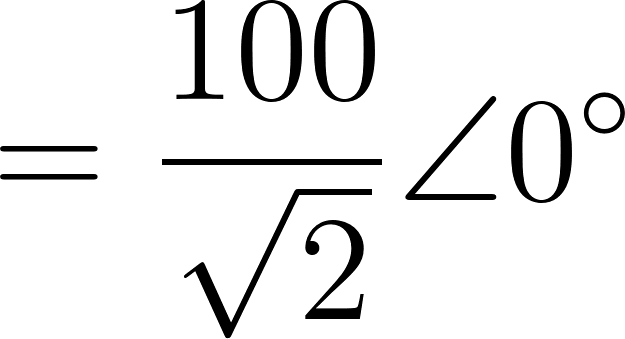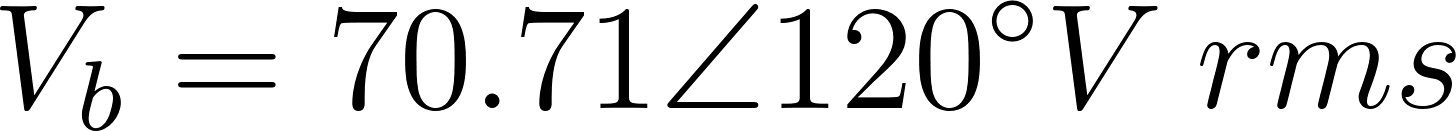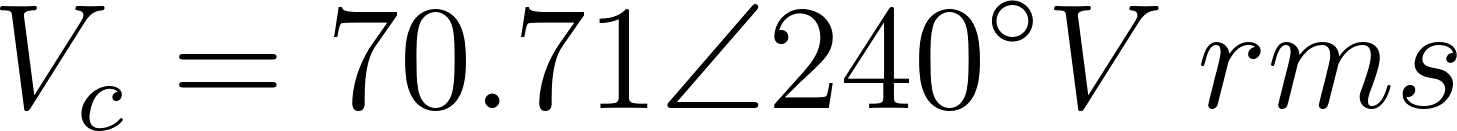Introduction To Electric Circuits - 9 Edition - Chapter 12.3 - Problem P12.3-5
Register Now

Join StudySoup

Get Full Access to Introduction To Electric Circuits - 9 Edition - Chapter 12.3 - Problem P12.3-59781118477502

# A balanced YY circuit is shown in Figure P 12.3-5. Find the average power delivered to

Introduction to Electric Circuits | 9th Edition

Problem P12.3-5

A balanced YY circuit is shown in Figure P 12.3-5. Find the average power delivered to the load.a b n N c A B C 60 mH 10 5 mH 20 60 mH 100 cos (377t + 240) 100 cos (377t +120) 10 5 mH 20 60 mH Source Line Load Figure P 12.3-5

Accepted Solution
Step-by-Step Solution:

Step 1 of 5

The circuit parameters in the frequency domain,Therefore,###### Chapter 12.3, Problem P12.3-5 is Solved

Step 2 of 5

Step 3 of 5

Unlock Textbook Solution